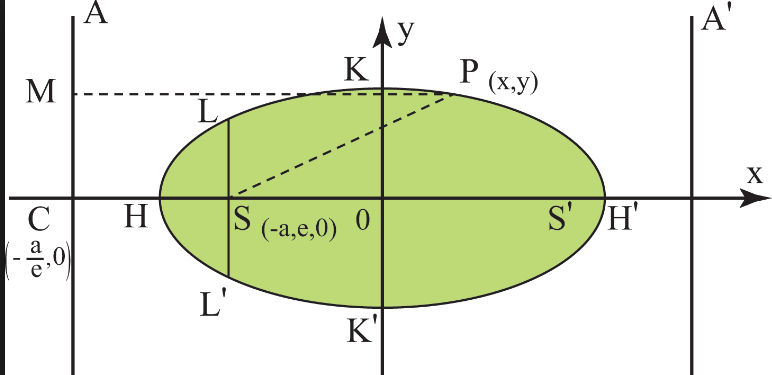Q

# Need clarity, kindly explain! If the length of the latus rectum of an ellipse is 4 units and the distance between a focusand its nearest vertex on the major axis is3/2units, then its eccentricity is :

If the length of the latus rectum of an ellipse is 4 units and the distance between a focus
and its nearest vertex on the major axis is
3/2
units, then its eccentricity is :

• Option 1)

• Option 2)

• Option 3)

• Option 4)

201 Views

As we have learned

Length of latus rectum of ellipse -

- wherein

For the ellipse

Eccentricity -

- wherein

For the ellipse

Sum of focal distance -

- whereinLength of LR

also

also

thus

thus

Option 1)

This is incorrect

Option 2)

This is correct

Option 3)

This is incorrect

Option 4)

This is incorrect

Exams
Articles
Questions